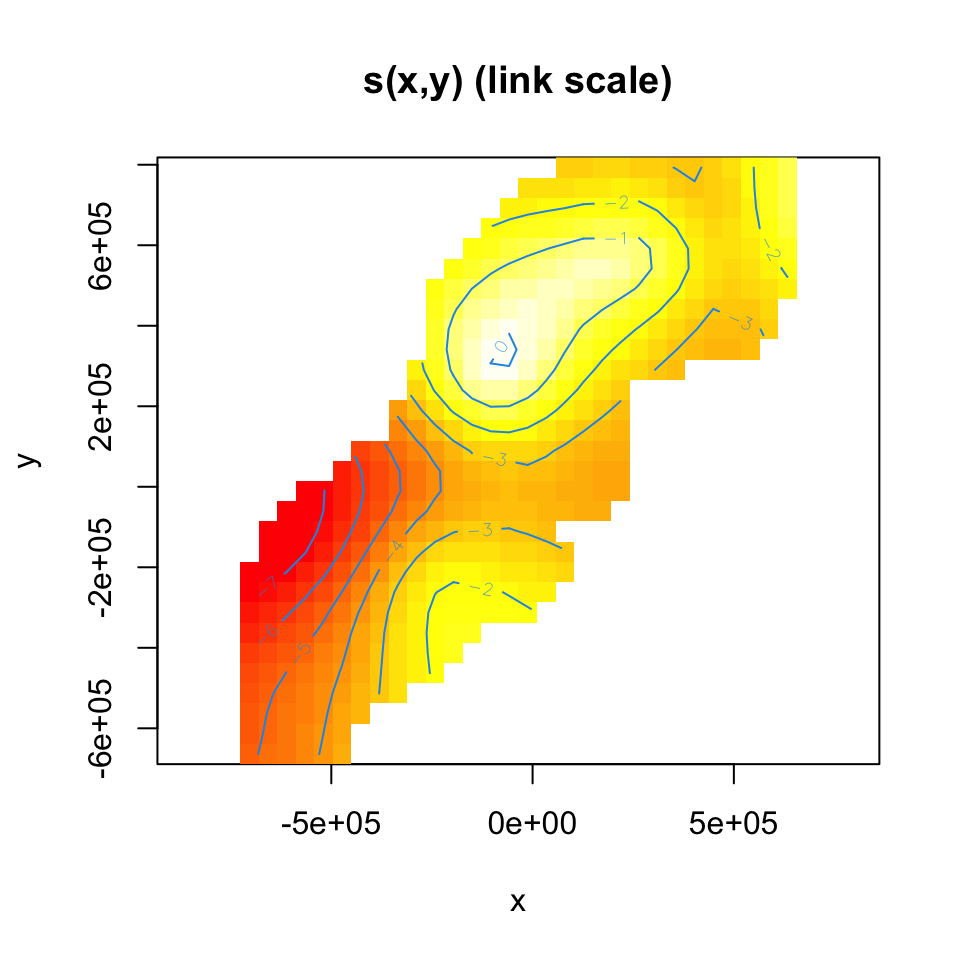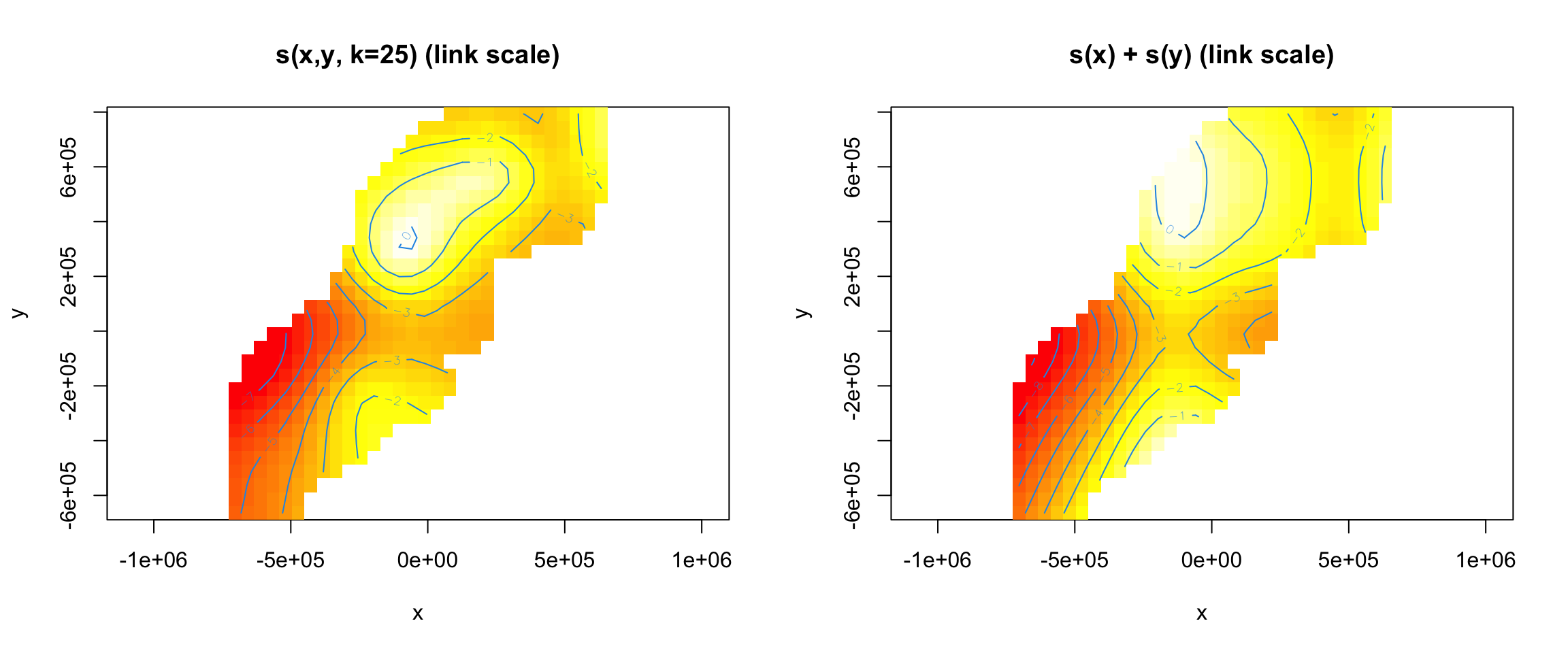## Aims

By the end of this practical, you should feel comfortable:

• Fitting a density surface model using `dsm()`
• Understanding what the objects that go into a `dsm()` call
• Understanding the role of the response in the `formula=` argument
• Understanding the output of `summary()` when called on a `dsm` object
• Increasing the `k` parameter of smooth terms to increase their flexibility

The example code below uses the `df_hn` detection function in the density surface models. You can substitute this for your own best model as you go, or copy and paste the code at the end and see what results you get using your model for the detection function.

In this practical, we’re just going to do basic comparisons looking at plots and `summary` output. We’ll get more into model comparison in later practicals.

Solutions are given between these horizontal lines with bold headers

The “solutions” presented here are not definitive, many modelling options are possible.

Here I’ll use a few different detection functions that I fitted in the first practical. To run the code in this file, you’ll first need to have run the first practical solution, to get the correct outputs.

## Load the packages and data

``library(Distance)``
``## Loading required package: mrds``
``````## This is mrds 2.2.3
## Built: R 4.0.2; ; 2020-08-01 10:33:56 UTC; unix``````
``````##
## Attaching package: 'Distance'``````
``````## The following object is masked from 'package:mrds':
##
##     create.bins``````
``library(dsm)``
``## Loading required package: mgcv``
``## Loading required package: nlme``
``## This is mgcv 1.8-33. For overview type 'help("mgcv-package")'.``
``## Loading required package: numDeriv``
``````## This is dsm 2.3.1.9007
## Built: R 4.0.2; ; 2020-10-01 05:44:34 UTC; unix``````
``````library(ggplot2)
library(knitr)``````

Loading sperm whale data again and the `RData` files where we saved our results:

``````load("spermwhale.RData")

## Pre-model fitting

Before we fit a model using `dsm()` we must first remove the observations from the spatial data that we excluded when we fitted the detection function – those observations at distances greater than the truncation.

``obs <- obs[obs\$distance <= df_hn\$ddf\$meta.data\$width, ]``

Here we’ve used the value of the truncation stored in the detection function object, but we could also use the numeric value (which we can also find by checking the detection function’s `summary()`).

(If we don’t do this, the DSM will still fit fine, we just get a warning.)

## Fitting DSMs

Using the data that we’ve saved so far, we can build a call to the `dsm()` function and fit out first density surface model. Here we’re only going to look at models that include spatial smooths.

Let’s start with a very simple model – a bivariate smooth of `x` and `y`:

``````dsm_nb_xy <- dsm(count~s(x, y, k=25),
ddf.obj=df_hn, segment.data = segs,
observation.data=obs,
family=nb())``````

Note again that we try to have informative model object names so that we can work out what the main features of the model were from its name alone.

We can look at a `summary()` of this model. Look through the summary output and try to pick out the important information based on what we’ve talked about in the lectures so far.

``summary(dsm_nb_xy)``
``````##
## Family: Negative Binomial(0.093)
##
## Formula:
## count ~ s(x, y, k = 25) + offset(off.set)
##
## Parametric coefficients:
##             Estimate Std. Error z value Pr(>|z|)
## (Intercept) -20.4863     0.2207  -92.84   <2e-16 ***
## ---
## Signif. codes:  0 '***' 0.001 '**' 0.01 '*' 0.05 '.' 0.1 ' ' 1
##
## Approximate significance of smooth terms:
##          edf Ref.df Chi.sq  p-value
## s(x,y) 13.91  17.55  66.36 1.05e-06 ***
## ---
## Signif. codes:  0 '***' 0.001 '**' 0.01 '*' 0.05 '.' 0.1 ' ' 1
##
## R-sq.(adj) =   0.08   Deviance explained = 34.9%
## -REML = 394.96  Scale est. = 1         n = 949``````

Things to look for:

• Was the bivariate smooth of space (`s(x, y)`) significant at the highest level?
• What was the effective degrees of freedom (EDF) for this model? How does that compare with the `k` we provided?
• Does the number of observations (`n=`) match the number of rows in `segs`?

Summary output

• From the `summary` output we can see that the bivariate term, `s(x,y)` is significant at the “0” level – that’s not too surprising given it’s the only term in the model though!
• The EDF for the model was about 14. We set `k=25` above, so we’re nowhere near using all of the degrees of freedom we gave the model. Note that we don’t need to reduce `k`, the penalty has done its job and reduced the wigglyness of the model accordingly.
• We see from the `summary` that `n = 949`, we can check the number of rows in the segment data:
``nrow(segs)``

and confirm that’s correct. These might not match if you have `NA` (“not available”) values in your segment data, for example if you have covariates that don’t have values (SST due to cloud for example). If there are `NA`s where values are needed, the whole row will be dropped. It’s usually worth checking this to make sure you’re fitting to the data that you think you are.

### Visualising output

As discussed in the lectures, the `plot` output is not terribly useful for bivariate smooths like these. We’ll use `vis.gam()` to visualise the smooth instead:

``````vis.gam(dsm_nb_xy, view=c("x","y"), plot.type="contour",Fitted surface (on link scale) for s(x,y)

Notes:

1. The plot is on the scale of the link function, the offset is not taken into account – the contour values do not represent abundance!
2. We set `view=c("x","y")` to display the smooths for `x` and `y` (we can choose any two variables in our model to display like this)
3. `plot.type="contour"` gives this “flat” plot, set `plot.type="persp"` for a “perspective” plot, in 3D.
4. The `too.far=0.1` argument displays the values of the smooth not “too far” from the data (try changing this value to see what happens).
5. `asp=1` ensures that the aspect ratio of the plot is 1, making the pixels square.
6. Read the `?vis.gam` manual page for more information on the plotting options.

### Setting basis complexity

We can set the basis complexity via the `k` argument to the `s()` term in the formula. For example the following re-fits the above model with a much smaller basis complexity than before:

``````dsm_nb_xy_smallk <- dsm(count~s(x, y, k=10),
ddf.obj=df_hn, segment.data = segs,
observation.data=obs,
family=nb())``````

Compare the output of `vis.gam()` for this model to the model with a larger basis complexity.

## Univariate models

Instead of fitting a bivariate smooth of `x` and `y` using `s(x, y)`, we could instead use the additive nature and fit the following model:

``````dsm_nb_x_y <- dsm(count~s(x)+ s(y),
ddf.obj=df_hn, segment.data = segs,
observation.data=obs,
family=nb())``````

Compare this model with `dsm_nb_xy` using `vis.gam()` and `summary()`.

Comparing to the univariate model

First taking a look at the summary:

``summary(dsm_nb_x_y)``
``````##
## Family: Negative Binomial(0.085)
##
## Formula:
## count ~ s(x) + s(y) + offset(off.set)
##
## Parametric coefficients:
##             Estimate Std. Error z value Pr(>|z|)
## (Intercept) -20.4301     0.2374  -86.07   <2e-16 ***
## ---
## Signif. codes:  0 '***' 0.001 '**' 0.01 '*' 0.05 '.' 0.1 ' ' 1
##
## Approximate significance of smooth terms:
##        edf Ref.df Chi.sq  p-value
## s(x) 5.171  6.302  22.59 0.001711 **
## s(y) 4.779  5.886  25.73 0.000444 ***
## ---
## Signif. codes:  0 '***' 0.001 '**' 0.01 '*' 0.05 '.' 0.1 ' ' 1
##
## R-sq.(adj) =  0.052   Deviance explained = 31.3%
## -REML = 395.86  Scale est. = 1         n = 949``````

we can see that the `s(x)` and `s(y)` terms have EDFs lower than the (default) `k=10` they were given. The combined EDFs are bigger than those for the model with `s(x,y)` – perhaps because these terms have a lot of work to do without being able to properly model the interaction. We can see this when plotting the `s(x) + s(y)` model alongside the `s(x,y)` model:

``````par(mfrow=c(1,2))
vis.gam(dsm_nb_xy, view=c("x","y"), plot.type="contour",
too.far=0.1, main="s(x,y, k=25) (link scale)", asp=1)

vis.gam(dsm_nb_x_y, view=c("x","y"), plot.type="contour",
too.far=0.1, main="s(x) + s(y) (link scale)", asp=1)``````We can also look at univariate plots of `s(x)` and `s(y)` for `dsm_nb_x_y`:

``plot(dsm_nb_x_y, pages=1)``